By introducing a new variable solve biquadratic equation:

$-{x}^{4}+277{x}^{2}-15876=0$

x1 =  9
x2 =  -9
x3 =  14
x4 =  -14

### Step-by-step explanation: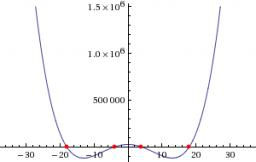Did you find an error or inaccuracy? Feel free to write us. Thank you!Tips to related online calculators
Looking for help with calculating roots of a quadratic equation?
Do you have a linear equation or system of equations and looking for its solution? Or do you have a quadratic equation?

## Related math problems and questions:

• Solve equationsolve equation: [(a2)+3]1/2 + [(a2)-3]1/2 = 5
• Completing squareSolve the quadratic equation: m2=4m+20 using completing the square method
• Three members GPThe sum of three numbers in GP (geometric progression) is 21 and the sum of their squares is 189. Find the numbers.
• Substitution method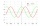Solve goniometric equation: sin4 θ - 1/cos2 θ=cos2 θ - 2Solve quadratic equation: 2x2+28x-550=0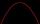Solve pure quadratic equation -5x2 +10 = 0.
• Logarithmic equation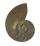Solve equation: log13(7x + 12) = 0
• Factors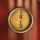Can the expression ? be factored into rational factors?
• Non linear eqsSolve the system of non-linear equations: 3x2-3x-y=-2 -6x2-x-y=-7
• Square root 2If the square root of 3m2 +22 and -x = 0, and x=7, what is m?
• RootsDetermine the quadratic equation absolute coefficient q, that the equation has a real double root and the root x calculate: 5x 2 +9x + q = 0
• Square 2Points D[10,-8] and B[4,5] are opposed vertices of the square ABCD. Calculate area of the square ABCD.
• EQ2Solve quadratic equation: 2x²- 2(x-7)²+5x=0
• The sumThe sum of the squares of two immediately following natural numbers is 1201. Find these numbers.Determine the numbers b, c that the numbers x1 = -1 and x2 = 3 were roots of quadratic equation: -3x 2 + b x + c = 0For the various digits A, B, C is true: the square root of the BC is equal to the A and sum B+C is equal to A. Calculate A + 2B + 3C. (BC is a two-digit number, not a product).Points A[9,9] and B[-4,1] are adjacent vertices of the square ABCD. Calculate the area of the square ABCD.